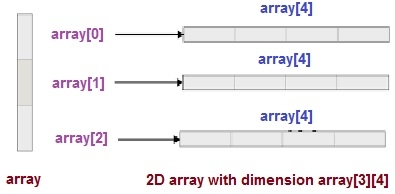# How do I declare a 2d array in C++ using new

A dynamic 2D array is basically an array of pointers to arrays. Here is a diagram of a 2D array with dimenation 3 x 4.## Algorithm

Begin
Declare dimension of the array.
Dynamic allocate 2D array a[][] using new.
Fill the array with the elements.
Print the array.
Clear the memory by deleting it.
End

## Example Code

Live Demo

#include <iostream>
using namespace std;
int main() {
int B = 4;
int A = 5;
int** a = new int*[B];
for(int i = 0; i < B; ++i)
a[i] = new int[A];
for(int i = 0; i < B; ++i)
for(int j = 0; j < A; ++j)
a[i][j] = i;
for(int i = 0; i < B; ++i)
for(int j = 0; j < A; ++j)
cout << a[i][j] << "\n";
for(int i = 0; i < A; ++i)
delete [] a[i];
delete [] a;
return 0;
}

## Output

0
0
0
0
0
1
1
1
1
1
2
2
2
2
2
3
3
3
3
3

Updated on: 30-Jul-2019

206 Views